Students can download Maths Chapter 4 Geometry Ex 4.2 Questions and Answers, Notes, Samacheer Kalvi 6th Maths Guide Pdf helps you to revise the complete Tamilnadu State Board New Syllabus, helps students complete homework assignments and to score high marks in board exams.

## Tamilnadu Samacheer Kalvi 6th Maths Solutions Term 1 Chapter 4 Geometry Ex 4.2

Question 1.
Use any number of the given dots to make different angles.
(i) An Accute angle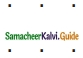(ii) An Obtuse Angle(iii) A Right Angle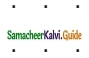(iv) A Straight Angle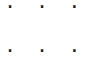Solution:
(i) An Accute angle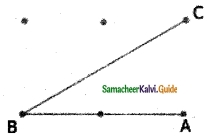(ii) An Obtuse Angle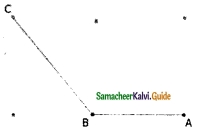(iii) A Right Angle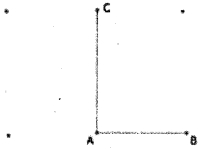(iv) A Straight Angle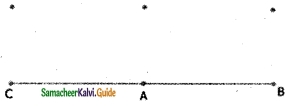Question 2.
Name the vertex and sides that form each angle.Solution:
(i) D, DE and DF
(ii) D, DE and DC
(iii) P, PQ and PR
(iv) S, SV and STQuestion 3.
Pick out the Right angles from the given figures.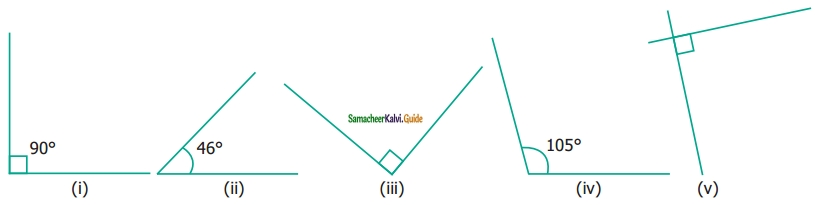Solution:
(i), (iii), (v)

Question 4.
Pick out the Accute angles from the given figures.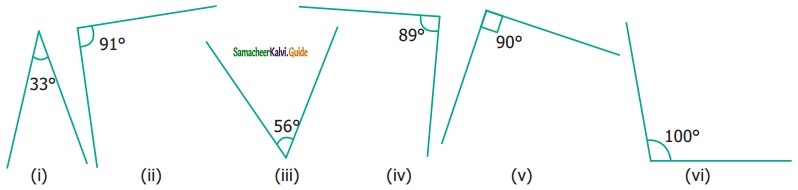Solution:
(i), (iii), (iv) are the Acute Angles.Question 5.
Pick out the Obtuse angles from the given figures.Solution:
(i) and (ii) are the Obtuse Angles.Question 6.
Name the angle in each figure given below in all the possible ways.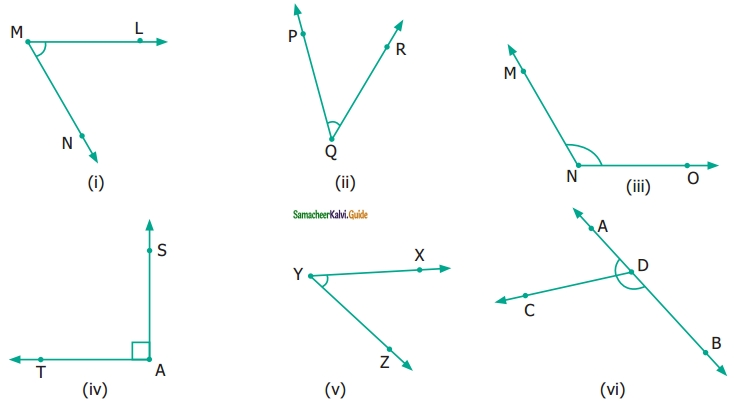Solution:
(i) ∠M or ∠LMN or ∠NML
(ii) ∠Q or ∠PQR or ∠RQP
(iii) ∠N or ∠MNO or ∠ONM
(iv) ∠A or ∠TAS or ∠SAT
(v) ∠Y or ∠XYZ or ∠ZYX
(vi) There are 3 angles in (vi)

• ∠ CDB or ∠BDC
• ∠D or ∠ADB or ∠BDAQuestion 7.
Say True or False.
(i) 20° and 70° are complementary.
(ii) 88° and 12° are complementary.
(iii) 80° and 180° are supplementary.
(iv) 0° and 180° are supplementary.
Solution:
(i) True
Hint: 20°+ 70° = 90°
(ii) False
Hint: 88° + 180° = 260° ≠ 1
(iii) False
Hint: 80° + 180° = 260° ≠ 1
(iv) True
Hint: 0° + 180° = 180°

Question 8.
Draw and label each of the angles.
(i) ∠NAS = 90°n
(ii) ∠BIG = 35°
(iii) ∠SMC = 145°
Solution: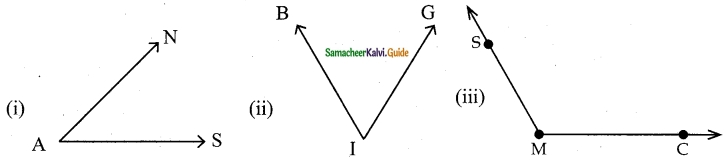Question 9.
Identify the types of angles shown by the hands of the given clock.Solution:
(i) Obtuse angle
(ii) Zero angle
(iii) Straight angle
(iv) Acute angle
(v) Right angle

Question 10.
Find the supplementary/complementary angles in each case.Solution: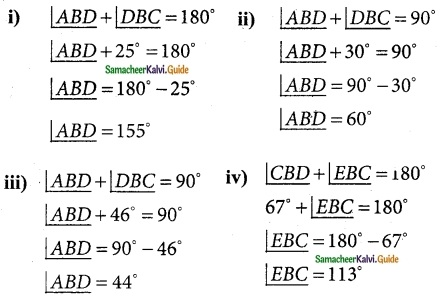Objective Type Questions

Question 11.
In this figure, which is not the correct way of naming an angle?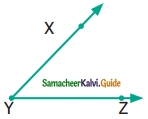(a) ∠Y
(b) ∠ZXY
(c) ∠ZYX
(d) ∠XYZ
Solution:
(b) ∠ZXYQuestion 12.
In this figure, ∠AYZ = 45. If point ‘A’ is shifted to point ‘B’ along the ray, then the measure of ∠BYZ is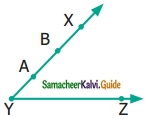(a) more than 45°
(b) 45°
(c) less than 45°
(d) 90°
Solution:
(b) 45°